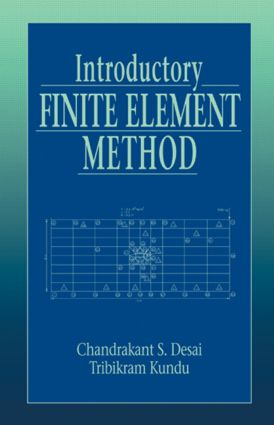# Introductory Finite Element Method

## 1st Edition

CRC Press

520 pages | 200 B/W Illus.

##### Purchasing Options:\$ = USD
Hardback: 9780849302435
pub: 2001-05-18
\$145.00
x
eBook (VitalSource) : 9781315274232
pub: 2017-12-19
from \$28.98

FREE Standard Shipping!

### Description

Although there are many books on the finite element method (FEM) on the market, very few present its basic formulation in a simple, unified manner. Furthermore, many of the available texts address either only structure-related problems or only fluid or heat-flow problems, and those that explore both do so at an advanced level.

Introductory Finite Element Method examines both structural analysis and flow (heat and fluid) applications in a presentation specifically designed for upper-level undergraduate and beginning graduate students, both within and outside of the engineering disciplines. It includes a chapter on variational calculus, clearly presented to show how the functionals for structural analysis and flow problems are formulated. The authors provide both one- and two-dimensional finite element codes and a wide range of examples and exercises. The exercises include some simpler ones to solve by hand calculation-this allows readers to understand the theory and assimilate the details of the steps in formulating computer implementations of the method.

Anyone interested in learning to solve boundary value problems numerically deserves a straightforward and practical introduction to the powerful FEM. Its clear, simplified presentation and attention to both flow and structural problems make Introductory Finite Element Method the ideal gateway to using the FEM in a variety of applications.

### Reviews

"This is an excellent addition to the available textbooks on finite element method…The mathematical formulations and fundamental concepts illustrated with simple examples and summaries or comments and plenty of exercises presented at the end of each chapter are other merits of the book…The book is highly recommended for undergraduate students and teachers in universities."

- Appl Mech Rev, Vol. 55, no. 1

"Intended for use… within and outside engineering disciplines, this book examines both stress-deformation analysis and flow (heat and fluid) applications…. offers an introduction to the finite element method…"

-Sci Tech Book News, Vol. 25, No. 3, September 2001

INTRODUCTION

Basic Concept

Process of Discretization

Principles and Laws

Cause and Effect

Review Assignments

STEPS IN THE FINITE ELEMENT METHOD

Introduction

General Idea

Introduction to Variational Calculus

Summary

ONE-DIMENSIONAL STRESS DEFORMATION

Introduction

Explanation of Global and Local Coordinates

Local and Global Coordinate System for the One-Dimensional Problem

Interpolation Functions

Relation Between Local and Global Coordinates

Requirements for Approximation Functions

Stress-Strain Relation

Principle of Minimum Potential Energy

Expansion of Terms

Integration

Direct Stiffness Method

Boundary Conditions

Strains and Stresses

Formulation by Galerkin's Method

Computer Implementation

Other Procedures for Formulation

Complementary Energy Approach

Mixed Approach

Bounds

Advantages of the Finite Element Method

ONE-DIMENSIONAL FLOW

Theory and Formulation

Problems

Bibliography

ONE-DIMENSIONAL TIME-DEPENDENT FLOW: Introduction to Uncoupled and Coupled Problems

Uncoupled Case

Time-Dependent Problems

One-Dimensional Consolidation

Computer Code

FINITE ELEMENT CODES: ONE AND TWO-DIMENSIONAL PROBLEMS

One-Dimensional Code

Philosophy of Codes

Stages

Explanation of Major Symbols and Arrays

User's Guide for Code DFT/C1DFE

Two-Dimensional Code

User's Guide for Plane-2D

Sample Problems for Plane-2D

User's Guide for Field-2D

Sample Problems for Field-2D

BEAM BENDING AND BEAM COLUMN

Introduction

Beam-Column

Other Procedures for Formulation

ONE-DIMENSIONAL MASS TRANSPORT

Introduction

Finite Element Formulation

References

Bibliography

ONE-DIMENSIONAL STRESS WAVE PROPAGATION

Introduction

Finite Element Formulation

Convection Parameter ux

Bibliography

TWO AND THREE DIMENSIONAL FORMULATIONS

Introduction

Two-Dimensional Formulation

Three-Dimensional Formulation

ONE-DIMENSIONAL STRESS WAVE PROPAGATION

Introduction

Finite Element Formulation

Boundary and Initial Conditions

Boundary Conditions

TWO-AND THREE-DIMENSIONAL FORMULATIONS

Introduction

Two-Dimensional Formulation

Triangular Element

Three-Dimensional Formulation

Tetrahedron Element

Brick Element

TORSION

Introduction

Finite Element Formulation (Displacement Approach)

Comparison of Numerical Predictions and Closed Form Solutions

Stress Approach

Hybrid Approach

Mixed Approach

Static Condensation

OTHER FIELD PROBLEMS: POTENTIAL, THERMAL, FLUID, AND ELECTRICAL FLOW

Introduction

Potential Flow

Finite Element Formulation

Stream Function Formulation

Thermal or Heat Flow Problem

Seepage

Electromagnetic Problems

Computer Code

TWO-DIMENSIONAL STRESS-DEFORMATION ANALYSIS

Introduction

Plane Deformations

Finite Element Formulation

Computer Code

MULTICOMPONENT SYSTEMS: BUILDING FRAME AND FOUNDATION

Introduction

Various Components

Computer Code

Transformation of Coordinates

APPENDIX 1: Various Numerical Procedures: Solution to Beam Bending Problem

APPENDIX 2: Solution of Simultaneous Equations

APPENDIX 3 Computer Codes

Each chapter also contains sections of problems and references

### Subject Categories

##### BISAC Subject Codes/Headings:
TEC009020
TECHNOLOGY & ENGINEERING / Civil / General
TEC009070
TECHNOLOGY & ENGINEERING / Mechanical